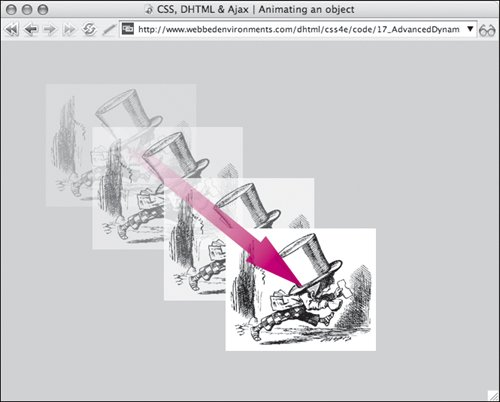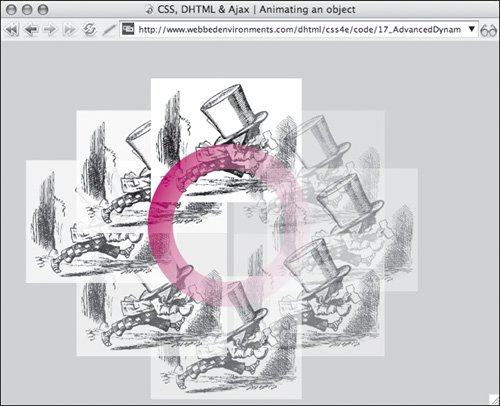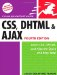# Animating an Object

When most people think about dynamic techniques, they don't think of simply moving objects from one point to another (see "Moving Objects from Point to Point" in Chapter 16), but of making objects slide across the screen from one point to another or along a curved path.

Using a function that runs recursively (see the previous section, "Making a Function Run Again"), you can make any object that has been positioned (see "Setting an Element's Position" in Chapter 7) seem to glide from one point to another.

#### Animating an Object in a Straight Line

For a straight line, the process of animation is relatively straightforward: Simply move the object incrementally horizontally and/or vertically step-by-step from its first position to its last position.

There is one small snag, though; if the horizontal and vertical distances the object has to move are not the same value, you'll need to adjust step movement to get a straight line. This is handled by calculating the slope of the angle between the two points, and using this value to adjust how far the object should be moved in a single step.

In this example (Figure 17.2), we set the initial starting point of our object, and then calculate each point along the path to its final position, moving it just a little bit with each step.

##### Figure 17.2. The Mad Hatter dashes across the screen.To animate an object in a straight line:

1.

`var animateSpeed = 5;`

Initialize the following global variables (Code 17.2):

##### Code 17.2. The startAnimate() function finds the initial left and top positions of the object and then triggers the animateObject() function to start the animation.

 [View full width]` CSS, DHTML & Ajax | Animating an object
`

• animateSpeed sets the increment the object is moved with each step. The larger the number, the slower the object slides, but the choppier the animation looks.

• object records the object's ID.

• fX records the final left position of the object.

• fY records the final top position of the object.

• cX records the current left position of the object.

• cY records the current top position of the object.

• dX keeps track of the amount the object has moved to the left while being animated.

• dY keeps track of the amount the object has moved from the top while being animated.

• stepX records how far the object should move horizontally for each step in the animation.

• stepY records how far the object should move vertically for each step in the animation.

• slope records the ratio of x to y, for the slant of the object's path from the starting position to its final position. This is used to calculate the x and y step values so that the object goes in a straight line between the two points.

2.

`function initAnimate(objectID, x, y){...}`

Add the function initAnimate() to your JavaScript. This function uses the ID of the object to locate it on the screen, sets the final x, y position of the object (fX and fY), calculates the current x,y position of the object (cX and cY), calculates the slope of the animation path, and then uses that to calculate how far the object should move horizontally and vertically for each step in the animation. Finally, this function runs the animateObject() function.

The mathematics in this function may look a bit complex at first, but it's really straightforward addition and/or subtraction. To allow for the final position being above, below, to the left, or to the right of the initial position, we have to add several conditionals.

3.

`function animateObject() {...}`

Add the function animateObject() to your JavaScript. This function checks to see if the object has moved past its final position. If not, it moves the object to its new position, calculates the next position it should be moved to by adding the step variables to the current position, recalculates the current position, and then runs the function again. If the object has moved to its final position, it is moved back slightly to compensate, and then the function ends.

4.

`#madHatter {...}`

Set up the CSS rule for your animated object with values for position and top and left positions.

5.

`onload="initAnimate('madHatter', 200, 200);"`

Add an event handler to the body element to trigger the function you created in Step 2, passing the function the ID of the object you want to animate and the final x,y position to which you want that object to move.

6.

`<div >...</div>`

Set up the object to be animated.

#### Animating an Object in a Circle

In many ways, a circular animation is easier to code than a straight line, because you don't need to keep track of the slope. Instead, simply feed the formula for the radius of the circle and the script takes it from there.

In this example (Figure 17.3), the cosine (Math.cos()) and sine (Math.sin()) methods are used to calculate the circumference with the given radius, creating a roughly circular path.

##### Figure 17.3. The Mad Hatter dashes around in a circle.To animate an object in a circle:

1.

`animateSpeed = 10;`

Initialize the following global variables (Code 17.3):

##### Code 17.3. The circular animation script calculates where the object should be displayed along the circumference of a circle, based on a radius that you initially feed it.

 [View full width]` CSS, DHTML & Ajax | Animating an object
`

• animateSpeed sets the amount of delay in the recursive running of the function. The larger the number, the slower the object slides, but the choppier the animation looks.

• object records the object's ID.

• cX records the current left position of the object.

• cY records the current top position of the object.

• fX records the final left position of the object.

• fY records the final top position of the object.

• next keeps track of the amount that the object has moved around the circular path.

• radius keeps track of the distance from the object to the center of the circle around which the object is being animated.

2.

`function initAnimateCircle(objectID, theRadius) {...}`

Add the function initAnimateCircle() to your JavaScript.

This function uses the ID of the object to locate it on the screen, finds the current x,y position of the object (cX and cY), and also stores this as the object's final x,y position (fX and fY). Finally, this function runs the animateObjectCircle() function.

3.

`function animateObjectCircle() {...}`

Add the function animateObjectCircle() to your JavaScript.

This function first checks to see if the object has made a full circle (in this example, 72 steps around the circumference). If not, the function calculates the next position of the object along the circumference of the circle:

`var nX = cX + (Math.cos(next * (Math.PI/36)) * radius); var nY = cY + (Math.sin(next * (Math.PI/36)) * radius);`

The function then moves the object, increases next by 1, and runs the function again. Once the function reaches 72, the object is reset to its initial (final) position. This ensures that the object is exactly positioned in case of any mathematical discrepancies that might offset it by a few pixels.

4.

`#madHatter {...}`

Set up the CSS rule for your animated object with values for position and top and left positions.

5.

`onload="initAnimateCircle('madHatter', 10);"`

Add an event handler to the body element to trigger the function you created in Step 2, passing the function of the ID of the object you want to animate and the radius of the circle around which you want to animate it.

6.

`<div >...</div>`

Set up the object to be animated.Tip

• The formula used to calculate the circumference of the circle could be replaced by any mathematical formulae to create a variety of curved paths.CSS, DHTML, and Ajax, Fourth Edition
ISBN: 032144325X
EAN: 2147483647
Year: 2006
Pages: 230

Similar book on Amazon

flylib.com © 2008-2017.
If you may any questions please contact us: flylib@qtcs.net